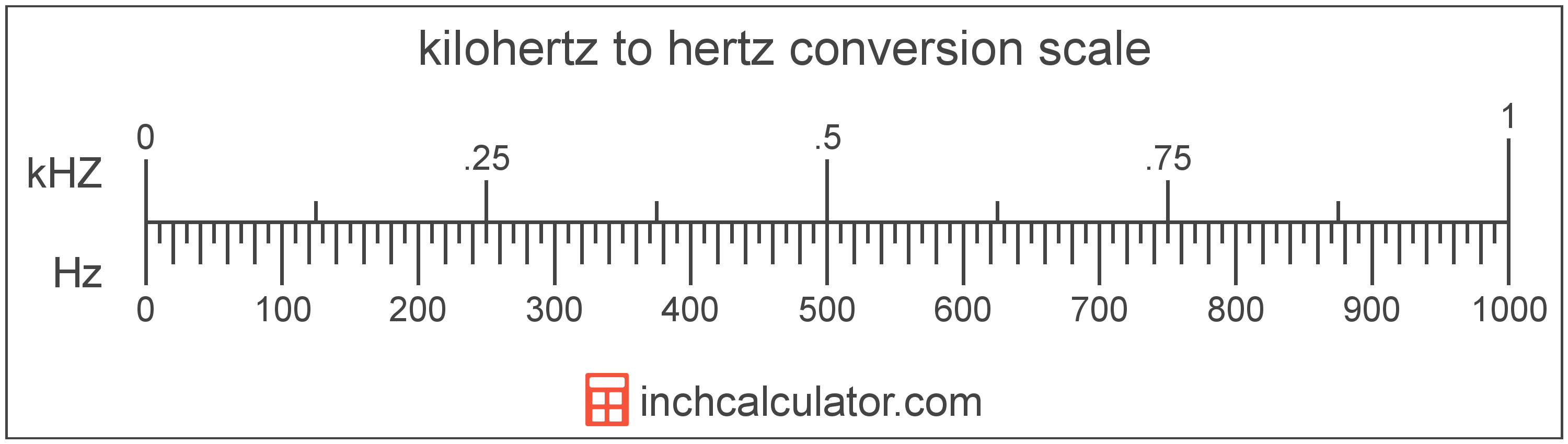# Kilohertz to Hertz Converter

Enter the frequency in kilohertz below to get the value converted to hertz.

Results in Hertz:1 kHZ = 1,000 Hz

Do you want to convert hertz to kilohertz?

## How to Convert Kilohertz to Hertz

To convert a measurement in kilohertz to a measurement in hertz, multiply the frequency by the following conversion ratio: 1,000 hertz/kilohertz.

Since one kilohertz is equal to 1,000 hertz, you can use this simple formula to convert:

hertz = kilohertz × 1,000

The frequency in hertz is equal to the frequency in kilohertz multiplied by 1,000.

For example, here's how to convert 5 kilohertz to hertz using the formula above.
hertz = (5 kHZ × 1,000) = 5,000 Hz### How Many Hertz Are in a Kilohertz?

There are 1,000 hertz in a kilohertz, which is why we use this value in the formula above.

1 kHZ = 1,000 Hz

## What is a Kilohertz?

Kilohertz is a measure of frequency equal to one thousand cycles per second.

The kilohertz is a multiple of the hertz, which is the SI derived unit for frequency. In the metric system, "kilo" is the prefix for thousands, or 103. Kilohertz can be abbreviated as kHZ; for example, 1 kilohertz can be written as 1 kHZ.

## What is a Hertz?

One hertz is formally defined as the frequency of one cycle per second.

The hertz is the SI derived unit for frequency in the metric system. Hertz can be abbreviated as Hz; for example, 1 hertz can be written as 1 Hz.

Frequency in hertz can be expressed using the formula:

Hz = Cycles / Time in Seconds

## Kilohertz to Hertz Conversion Table

Table showing various kilohertz measurements converted to hertz.
Kilohertz Hertz
0.001 kHZ 1 Hz
0.002 kHZ 2 Hz
0.003 kHZ 3 Hz
0.004 kHZ 4 Hz
0.005 kHZ 5 Hz
0.006 kHZ 6 Hz
0.007 kHZ 7 Hz
0.008 kHZ 8 Hz
0.009 kHZ 9 Hz
0.01 kHZ 10 Hz
0.02 kHZ 20 Hz
0.03 kHZ 30 Hz
0.04 kHZ 40 Hz
0.05 kHZ 50 Hz
0.06 kHZ 60 Hz
0.07 kHZ 70 Hz
0.08 kHZ 80 Hz
0.09 kHZ 90 Hz
0.1 kHZ 100 Hz
0.2 kHZ 200 Hz
0.3 kHZ 300 Hz
0.4 kHZ 400 Hz
0.5 kHZ 500 Hz
0.6 kHZ 600 Hz
0.7 kHZ 700 Hz
0.8 kHZ 800 Hz
0.9 kHZ 900 Hz
1 kHZ 1,000 Hz

## References

1. National Institute of Standards and Technology, SI Units - Time, https://www.nist.gov/pml/owm/si-units-time